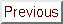# XMM-Newton Users HandbookNext: 6 Analysing XMM-Newton data Up: XMM-Newton UHB Previous: 4.6.3 Slew observations

# 5 Proposal Submission and Optimisation

The proposal submission process for XMM-Newton is divided into two phases:

In response to the call, principal investigators (PIs) are asked to provide the scientific justification and some basic source and observing parameters, like coordinates, observation duration and observing mode of the prime instrument. This is done using the RPS tool developed by HEASARC.

During the second phase of the process, the PIs of successful proposals must provide the exposure parameters in full detail via the Remote Proposal Submission software (XRPS). After submission each proposal undergoes a technical optimisation (the so called ``proposal enhancement'') by SOC personnel. Note that it is the proposer's responsibility to ensure that the target coordinates (and, if necessary, the spacecraft position angle) are correct.

The technical optimisation of the proposals will consider the following points:

• EPIC

1. Expected X-ray counts

Calculating the expected X-ray counts for the specified target for each filter of each EPIC instrument.

2. Expected optical count rate

Calculating the expected optical counts for each specified target for each filter of each EPIC instrument.

3. Telemetry bandwidth

Calculating the expected telemetry bandwidth for each exposure.

4. Science parameters

Calculating for each exposure:

• Expected pile-up
• Counts per frame

• RGS

1. Expected X-ray count rate

Calculating the expected X-ray counts for each specified target for each RGS instrument.

2. Telemetry bandwidth

Calculating the expected telemetry bandwidth for each exposure.

3. Science parameters

Estimating for each exposure, from the expected X-ray count rate, if pile-up may be present.

• OM

1. Count rate estimation

Calculating the counts expected in each window (either imaging or fast) of each OM exposure.

2. Science windows

• Calculating the size in detector pixels of each science window defined by the PI.

• Calculating the number of memory windows required to enclose the specified science windows and their details (position and size), and checking that the proposed configuration is allowed.

3. Bright objects

For each observation indicating:

• Whether the observation is possible with the filters specified by the PI.

• Which filters are allowed.

PIs will always be informed about the outcome of the evaluation described above. All changes of observation parameters have to be confirmed explicitly by the PIs.

This is a pre-condition for an observation to be included in the scheduling system (cf.
http://www.cosmos.esa.int/web/xmm-newton/proposal-enhancement).Next: 6 Analysing XMM-Newton data Up: XMM-Newton UHB Previous: 4.6.3 Slew observations
European Space Agency - XMM-Newton Science Operations Centre